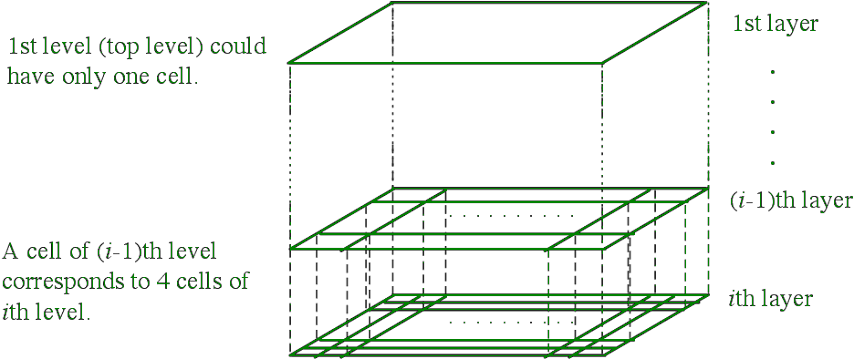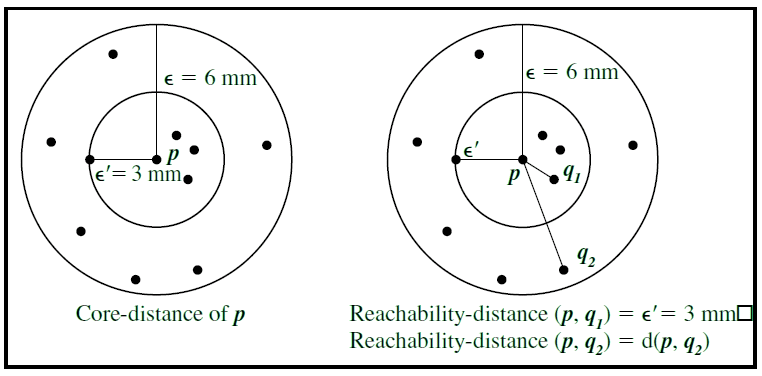GeeksforGeeks App
Open AppBrowser
Continue

# Difference between STING and OPTICS

The method of identifying similar groups of data in a data set is called clustering. Entities in each group are comparatively more similar to entities of that group than those of the other groups.

STING (Statistical Information Grid Clustering Algorithm) and OPTICS (Ordering Point To Identify Clustering Structure Clustering Algorithm) are clustering algorithms used in Unsupervised Learning. They are machine learning techniques which are used to club the given input data points into clusters or groups on the basis of their attributes. STING is grid-based clustering algorithm while OPTICS is a density-based clustering algorithm. Clustering

These are some differences between STING and OPTICS.

STING Algorithm:

1. Determine a layer, to begin with.
2. For each cell of this layer, we calculate the confidence interval (or estimated range) of probability that this cell is relevant to the query.
3. From the interval calculate above, we label the cell as relevant or not relevant.
4. If this is the bottom layer, then end the process.
5. We go down the hierarchy structure by one level. Go to Step 2 for those levels that form the relevant cells of the higher-level layer.

STING Hierarchy Diagram :OPTICS Algorithm:
Core distance of a point P is the smallest distance such that the neighborhood of P has atleast minPts points.
Reachability distance of p from q1 is the core distance ( ε’ ).
Reachability distance of p from q2 is the euclidean distance between p and q2.My Personal Notes arrow_drop_up